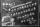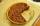# Lengths of the pool

Miguel swam 6 lengths of the pool. Mat swam 3 times as far as Miguel. Lionel swam 1/3 as far as Miguel. How many lengths did mat swim?

Result

c =  2

#### Solution:Leave us a comment of example and its solution (i.e. if it is still somewhat unclear...):Math student
If Kara cuts 2 pounds of cheese into blocks that each weigh
1
3
pound, how many blocks of cheese will she make?
blocksMath student
I hate that they don't have a word problem section too#### To solve this verbal math problem are needed these knowledge from mathematics:

Need help calculate sum, simplify or multiply fractions? Try our fraction calculator.

## Next similar examples:

1. Fractions 4How many 2/3s are in 6?
2. CakesOn the bowl were a few cakes. Jane ate one-third of them, Dana ate a quarter of those cakes that remained. a) What part (of the original number of cakes) Dana ate? b) At least how many cakes could be (initially) on thebowl?
3. Passenger boatTwo-fifths of the passengers in the passenger boat were boys. 1/3 of them were girls and the rest were adult. If there were 60 passengers in the boat, how many more boys than adult were there?
4. Scouts 44/7 of the students in a school are boys. If 3/8 of the boys are scouts, how many scouts are there in a school of 1878 students?
5. In fractionsAn ant climbs 2/5 of the pole on the first hour and climbs 1/4 of the pole on the next hour. What part of the pole does the ant climb in two hours?
6. Fraction to decimalWrite the fraction 3/22 as a decimal.
7. Mixed2improperWrite the mixed number as an improper fraction. 166 2/3
8. Fraction and a decimalWrite as a fraction and a decimal. One and two plus three and five hundredths
9. ZdeněkZdeněk picked up 15 l of water from a 100-liter full-water barrel. Write a fraction of what part of Zdeněk's water he picked.
10. Write 3Write a real world problem involving the multiplication of a fraction and a whole number with a product that is between 8 and 10 then solve the problem
11. Equation 20In given equation: 8/9-4/5=2/9+x, find x
12. HusseinHussein owns 450000. He spent at the bookstore 2 over 9 to buy some books and tales. He paid 3 over 5 of his money to buy his math book. Then he paid his money to buy stories diagram ; a. Calculate the remaining amount of money with Hussein? b. Hussein l
13. Product of two fractionsProduct of two fractions is 9 3/5 . If one of the fraction is 9 3/7. Find the other fraction.
14. Pie IIVili ate three pieces of pie. If it pieces is 1/8 how much pie did he eat?
15. TeacherTeacher Rem bought 360 pieces of cupcakes for the outreach program of their school. 5/9 of the cupcakes were chocolate flavor and 1/4 wete pandan flavor and the rest were vanilla flavor. How much more chocolate flavor cupcakes than vanilla flavor?
16. Fractions 3Calculate 1/9 of 27:
17. Pizza 5You have 2/4 of a pizza and you want to share it equally between 2 people how much pizza does each person get?